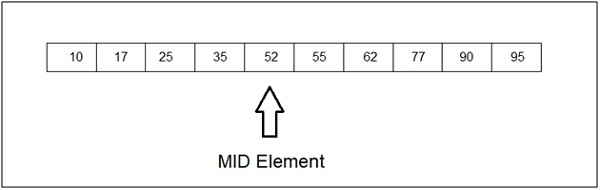# Binary search in C#

Binary search works on a sorted array. The value is compared with the middle element of the array. If equality is not found, then the half part is eliminated in which the value is not there. In the same way, the other half part is searched.

Here is the mid element in our array. Let’s say we need to find 62, then the left part would be eliminated and the right part is then searched −These are the complexities of a binary search −

 Worst-case performance O(log n) Best-case performance O(1) Average performance O(log n) Worst-case space complexity O(1)

## Example

Let us see the method to implement the binary search −

public static object BinarySearchDisplay(int[] arr, int key) {
int minNum = 0;
int maxNum = arr.Length - 1;

while (minNum <=maxNum) {
int mid = (minNum + maxNum) / 2;
if (key == arr[mid]) {
return ++mid;
} else if (key < arr[mid]) {
max = mid - 1;
}else {
min = mid + 1;
}
}
return "None";
}